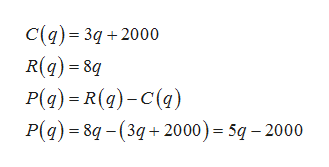A company has a cost function C(q) = 3q + 2000 dollars and revenue function R(q) = 8q dollarsFind the equation of the profit function P(q) _____________________________.  a.P(q) = 3q + 2000 b.P(q) = 5q + 2000 c.P(q) = 11q – 2000 d.P(q) = 5q – 2000 e.None of these

Question

A company has a cost function C(q) = 3q + 2000 dollars and revenue function R(q) = 8q dollars

Find the equation of the profit function P(q) _____________________________.

 a. P(q) = 3q + 2000 b. P(q) = 5q + 2000 c. P(q) = 11q – 2000 d. P(q) = 5q – 2000 e. None of these
Step 1

We know that , profit is the difference between revenue and cost

Step 2

So profit function =revenue f...help_outlineImage TranscriptioncloseC(q) 3q 2000 R(q)8q P(a) R(g)-C() P(q) 8q-(32000)= 5q -2000 fullscreen

Want to see the full answer?

See Solution

Want to see this answer and more?

Our solutions are written by experts, many with advanced degrees, and available 24/7

See Solution
Tagged in

Other